﻿ A Real-time Operation Scheme of Microgrids with Distributed Generation in Electricity MarketsPublications are Open
Access in this journal
Article Versions
Export Article
• Normal Style
• MLA Style
• APA Style
• Chicago Style
Research Article
Open Access Peer-reviewed

### A Real-time Operation Scheme of Microgrids with Distributed Generation in Electricity Markets

Nguyen Minh Y
American Journal of Electrical and Electronic Engineering. 2017, 5(5), 189-194. DOI: 10.12691/ajeee-5-5-4
Published online: December 08, 2017

### Abstract

This paper addresses the operation problem of microgrids under market environments. Considering the flexibility of DG sources in operation as well as the availability of market prices, microgrid operators can improve the profit in electricity markets by properly adjusting DG units in real-time corresponding to the price signal which is updated few-hour ahead. Starting with the basic formulation of unit commitments, the paper derives the optimality condition of DG units, i.e., generation levels for maximizing the profit assuming all units are being On (committed). If the capacity constraint results in an excess of the marginal cost to the locational market price, the unit will be considered to be Off. Taken into account the cost associated with switching On/Off the unit, an economic comparison will determine the optimal decision of whether to turn the unit On/Off or remain its current state. The proposed operation scheme is examined in a case study to illustrate its effectiveness with different scenarios of the market price.

### 1. Introduction

Distributed Generation (DG) encompasses various prime movers such as small gas-turbine, fuel cell (FC), photovoltaics (PV) and small wind turbine (WT), etc. which has been considered as an important source of power systems in future 1, 2. Particularly, DG units can be integrated in coordination with energy storage devices and loads to improve the quality and reliability of power supply while reduce the negative impact on the local network; this is called Microgrid 2, 3, 4. In addition, the restructuring of electric power industry, and consequently the implementation of electricity markets have allowed small, independent enterprises to build and operate their own power source for profits. This means, based on the market price, the electric company can control the generating unit: Which will be On/Off, how much to generate, etc. in each hour to improve the profit 5, 6, 7. It is thanks to Smartgrid technologies that allow the electricity price to be informed to all market participant few-hours in advances. Therefore, it is needed the operation problem should be resolved in real-time in accordance with the time basis of the market price.

There are many study efforts have been paid to address the operation problem of microgrids and DG sources. In 8, a centralized controller for microgrid operation is presented; the algorithm is to determine the optimal bidding of DG units and power exchanged with the main distribution grid by “priority-list” methods that maximizes the profit in the day-ahead (spot) electricity market. In 9, 10, the same problem is resolved by multi-agent systems in which the microgrid is divided into agents; each of them will compete for its own profit based on an auction algorithm; by this means, the operation of microgrids is optimized in the whole. The optimization of microgrids with both thermal and electrical loads is addressed in 11; the objective is to reduce the fuel cost by the optimal droop sharing (marginal cost curve) between DG units. In 12, the design and unit commitments of microgrids are formulated and solved by linear programming (LP) method; the formulation is developed in dynamic programming (DP) framework and solved by DP backward algorithm in 13. In the above-mentioned work, the operation schemes are based on large-scale, non-linear, mix-integer combinatorial optimization problems. The solution of such the problem is usually suffered from a high cost of computation and time requirement and cannot be done in real-time.

In this paper, we introduce a fast, decoupled operation scheme for microgrids in real-time electricity markets. First, the scheme starts with a sub-problem to determine the optimality condition of DG units assuming all units are being On: the marginal cost (MC) equal to the locational market price (LMP). There may exist sometimes that the unit makes negative profit (MC > LMP) because of the capacity constraint, the unit needs to be considered turning Off. In this case, a comparison is made between the saving in turning the unit Off versus the start-up and shut-down cost; as a result, the optimal On/Off decision as well as the generation level can be determined which give the maximum profit to the microgrid operator. Since only few moments in a day that a unit can make negative profit, the scheme can be applied in real-time corresponding to the time basis of the market price.

The remainder of the paper is organized as follows. Section 2 is for the notation and its meaning in the paper. Section 3 is the problem formulation with the objective function is maximizing the total profit of microgrids in electricity markets. Section 4 presents the proposed operation scheme with two solution stages: (1) determine the optimal generation with all units are On, (2) consider the negative-profit unit to be switched Off. Section 5 is the case study where the proposed scheme is applied to solve the optimal operation of microgrids. Finally, the significant points are summarized in Conclusion.

### 2. Notations

The notation used throughout the paper is stated below.

Indexes:

i: DG unit index

t: time interval (hour) index

N: number of DG units

T: scheduling period, [hour]

Constants and variables:

ρt: electricity price at hour t, [\$/MWh]

uit: commitment of DG unit i at hour t (1 means On, 0 means Off)

xit: On/Off state of DG unit i at hour t (hours has been On or Off)

PDt: electrical demand at hour t, [MWh]

PSt electricity purchased from the market at hour t, [\$/h]

PGi: power output of DG unit i at hour t, [MW]

PLt: power loss of the system at hour t, [MW]

Ci: fuel cost of DG unit i [\$/MWh]

Si: start-up cost of DG unit i [\$]

tminup,i: minimum-up time of DG unit i, [hour]

tmindn,i: minimum-down time of DG unit i, [hour]

ai,bi,ci: fuel cost coefficients of DG unit i, [\$/MW2], [\$/MW] and [\$], respectively

αi: start-up cost coefficient of DG unit i associated with others, [\$]

βi: start-up cost coefficient of DG unit i associated with cooling process, [\$]

τi: cooling time constant of DG unit i, [hour]

λt: Lagrangian multiplier at hour t

### 3. Mathematical Formulation

Unlikely the traditional generation source, e.g., coal-fired power plants which is working online most of its lifetime (except the time for repair and maintenance), DG units are more flexible in operation: the cost and time in starting and shutting process are not so large, allowing DGs to be turned On/Off even in a single day. Therefore, the microgrid operator can improve profits by properly responding to the markets price as expressed in Figure 1 13.

• Figure 1. The proposed operation scheme of microgrids

The objective function is maximizing profits over the pre-given market price. It can be expressed mathematically as follows.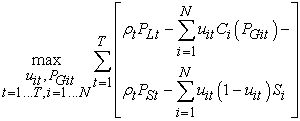(1)

Subject to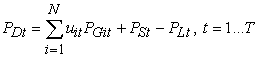(2)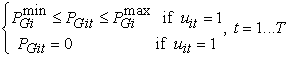(3)(4)(5)(6)

In equation (1), the first term is the revenue from providing electricity to the customer (the price, ρt, times quantity of consumption, PLt); the second term is the fuel cost of DG units which is defined as a quadratic function of the generation, PGit; the third term is the purchasing cost of electricity from the market (the price, ρt, times quantity purchased, PSt); and the last term is the start-up cost, i.e., the cost associated with the starting process of DG units. Equation (2) is the capacity limits of DG units: if the unit is On, it is needed to maintain the generation output within a certain range (min-max limits). Equation (3) shows the balancing constraint of supply and demand: the generation of DG units and purchasing from the market need to satisfy the customer’s demand. Equation (4) is the well-known modelling of the fuel cost as a quadratic function of the output.

Equation (5) shows the constraint of unit commitments which is associated with the technical and economical properties of thermal generating units: the unit must stay in On/Off state for a certain time before switching Off/On, respectively; it is called minimum-up and minimum-down time. Equation (6) is the transition of On/Off state of generating units: the positive and negative value means the unit have been On/Off for the time duration equal the absolute value of the On/Off state.

### 4. The Proposed Operation Scheme

As aforementioned, the above formulation is a large-scale, highly nonlinear and mix-integer optimization problem. The extract solution of such the problem requires a detail enumeration of all possible state which increases exponentially as the number of generating units; consequently, a huge computation and running time are involved. There is a fact that a generator in power systems is usually planned to work most of its lifetime. Only few moments of a day the generator needs considered to be Off: the generator make a negative profit. Turning On/Off a generator is usually suffered from an extra cost, therefore, a comparison between the On/Off options needs to be performed to determine the best solution for the operation problem. The proposed scheme consists of two functions: (1) pre-determine the optimal solution assuming all units are On, (2) compare the economic performance of On/Off options when the profit is negative and choose the better one.

Pre-determined generation level

Assuming all DG units are being On all the time, the problem can be decomposed into sub-problems that optimize the microgrid operation in each hour, independently.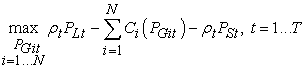(7)

Subject to(8)(9)

Apply Lagrangian multiplier to relax the equality constraint, the problem becomes maximizing the Lagrangian function as follows.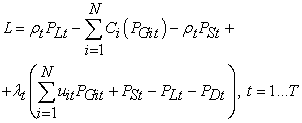(10)

To find the maximum point of the Lagrangian function, take derivatives with respect to the power output, PGit, and power purchased, PSt, to be zero:(11)(12)

The Lagrangian multiplier can be expressed in terms of the market price from equation (12) and substitute into (11), we have:(13)

Define the locational factor of DG unit i as follows.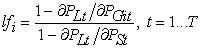(14)

Then, we have the optimality condition of DG units in electricity markets as follows.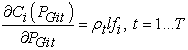(15)

Equation (15) shows that the output of DG units should be adjusted so that the marginal cost (MC) equal the locational market price (LMP), i.e., impact of the market price to the DG unit weighted by a locational factor, lf. Substitute the fuel cost in equation (4) into (15), we can obtain the optimal generation of DG units as follows.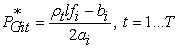(16)

Equation (16) shows the optimality condition of DG units if being On: it depends on the market price, fuel cost and the location of the unit in the system. It is noted that the output of DG units is limited by the capacity setup. If the result of equation (16) goes out of the limits, it will take the upper or lower boundary value as solutions. Therefore, the optimal generation of unit i at hour t can be rewritten as follows.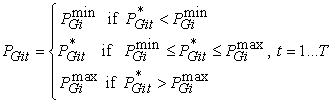(17)

Compare the On/Off Decision

In the above, we have derived the optimal generation of DG units if being On all the time. It is, of course, not always the desired solution, but giving us information when the DG unit need considered to be Off. That is when the unit makes negative profits, or in other words, MC is greater the LMP. If this is the case, an economic comparison between being On and Off of the unit need to be performed. It is noted that switching off is associated with an extra cost, i.e., start-up cost which is modelled as follows.(18)

The flow-chart algorithm of the proposed operation scheme is presented in the following figure.

• Figure 2. The flow-chart algorithm of the proposed operation scheme

### 5. Case Study

In this case study, we will apply the proposed scheme to an illustrative example to determine the optimal operation of microgrids in real-time. Suppose that the microgrid consists of five DG units and operate in a real-time electricity markets. The economic and technical properties of DG units are given in the below table.

For simplicity in analyzing the result in this example, we assume all the DG units have the same capacity limits (400 to 1000 kW); in which, the first two units have an identical cost function, and so do the last three ones. The minimum-up, minimum-down time are also the same. The locational factors are slightly different and greater than one meaning that the power loss caused by DG units is smaller than that of purchasing electricity from the market.

The electricity price is referred from the well-known PJM energy market  and modified by an amount to have the price signal in three cases: High, medium and low price scenarios in Case 1, 2 and 3, respectively [Figure 3]. The algorithm is coded in Matlab software, the optimal operation of the microgrid in three cases is expressed in the following.

• Figure 3. The electricity price in Case 1, 2 and 3

Figure 4 shows the optimal operation of the microgrid: the generation of DG units (P1-P5) and electricity purchased from the market (PS) in Case 1. It can be seen that the output of DG units is properly varying with the change of the market price to keep the MC of DG units equal to the LMP: the generation is low when the price is in valley (00:00-05:00) and increase as the price goes up (05:00-08:00); in (07:00-10:00) the unit 1, 2 reaches its maximum capacity limit and so do the unit 3, 4 and 5 in (06:00-12:00); then, the generation start to reduce because the price decreases (10:00-17:00); and the process continues the same in the following hours. It is worth noting that the electricity price is high in this case, making all DG units profitable when operating in the market; thus, the DG units are being On all the time. The identical cost function DG units have slightly different generation because of the locational factor resulting from their location in the network. The green-, circle-marked line shows the electricity purchased from the market to supply the customer’s demand.

• Figure 4. The optimal operation of the microgrid in Case 1

Figure 5 shows the optimal operation of the microgrid: the generation of DG units (P1-P5) and electricity purchased from the market (PS) in Case 2. It can be seen that the unit 1, 2 are turned Off sometimes while the unit 3, 4 and 5 are remained On all the time. It is because in this case the electricity price is lower compared to Case 1, making the unit 1, 2 suffered from negative profits if being On some hours when the price is in valley. The comparison shows that it is better to turn the unit 1, 2 Off and pay the starting-up and shutting-down cost rather than remaining On in these hour. The unit 3, 4 and 5 on the other hand have different (lower) fuel cost, they can make profit even when the price is in valley in this case. Thus, they are remained On all the time.

• Figure 5. The optimal operation of the microgrid in Case 2

Figure 6 shows the optimal operation of the microgrid: the generation of DG units (P1-P5) and electricity purchased from the market (PS) in case 3. In this case, the market price is even lower than Case 2, making all DG units suffered from negative profit when the price is in valley. Therefore, all units are supposed to be Off around (00:00-05:00) and (14:00-19:00); in these hours, it is better to purchase electricity from the market to serve all the customer’s demand because of the lower price. The effectiveness of the proposed scheme compared to the operation when the unit is On is expressed in Table 2.

• Figure 6. The optimal operation of the microgrid in Case 3

In Case 1, because of the high price of electricity all the units are making profits, no unit needs to be Off. Thus, the optimal operation of the proposed scheme is the same as if all the units are On, consequently no saving is received in this case. However, when the price is lower (Case 2 and 3), some units (the unit 1, 2 in Case 2 and all the units in Case 3) are making negative profit if being On sometimes. It is more economical if these units are turned Off in the negative-profit hours. Thus, the proposed operation scheme results in slight and significant saving in Case 2 and 3, respectively. The detail is presented in Table 2.

### 6. Conclusion

In this paper, we have presented a real-time operation scheme of microgrids in electricity market. Taking the flexibility and DG sources in operation as well as the information of the electricity price which is sent to all market participants few-hour ahead of time, the problem is to determine the On/Off decision and generation level of DG units and the electricity purchased from the market which maximizes the profit in overall the pre-informing price period. To solve the problem in real-time and avoid its large-scale, mix-integer, nonlinear property, the algorithm is divided into two stages: (1) pre-determine the optimal operation of all the units are On, and (2) if the unit makes a negative profit, compare the economics of On/Off decision and choose the best one. The proposed scheme has been successfully applied in case study to determine the optimal operation of a microgrid in three scenarios of the market price.

### Acknowledgements

This work is supported by Thai Nguyen University of Technology as a part of its project on Smartgrid and distributed generation.

### References

  Ackermann T., Andersson G., Soder L., “Distributed generation: A definition,” Electric Power System Research, 57(1): 195-204, 2001. In article View Article  Lasseter H. R., “Microgrid and distributed generation,” Journals of Energy Engineering, 133(1): 144-150, 2007. In article View Article  Piagi P. Robert, Lasseter H. R., “Autonomous control of microgrid,” Proc. IEEE Power Engineering Society General Meeting, Moreal, Canada, pp.1-8, 2006. In article View Article  Katiraei F., Iravani R., Hatziargyriou N., Dimeas A., “Microgrid management,” IEEE Power and Energy Magazine, 6(3): 54-65, 2008. In article View Article  Eric Allen and Marija Ilic, Price-based commitment decisions in the electricity market, Springer, 2006. In article View Article  Minh Y Nguyen, Yong T. Yoon, “Optimal scheduling and operation of battery/wind generation systems in response to real-time market prices,” IEEJ Transactions on Electrical and Electronic Engineering, 9(1): 129-135, 2014. In article View Article  Minh Y Nguyen, Yong T. Yoon, “Battery modelling for economic operation of energy storage systems in electricity markets,” International Review of Electrics Engineering, 8(3): 1000-1007, 2013. In article View Article  Antonis G. Tsikalakis, Nikos D. Hatziargyriou, “Centralized control for optimizing microgrids operation,” IEEE Transactions on Energy Conversion, 23(1): 241-248, 2008. In article View Article  Dimeas L., Hatziargyriou N. D., “Operation of a multiagent system for microgrid control,” IEEE Transaction on Power Systems, 20(3): 1447-1455, 2005. In article View Article  Hatziargyriou N., Dimeas A., Tsikalakis G., Lopes P., Karniotakis G., “Management of microgrid in market environment,” International Conference on Future Power System, Amsterdam, Netherlands, Nov. 2005. In article View Article  Hernadez C. Aramburon, T. C. Green, N. Mugniot, “Fuel consumption minimization of a microgrid,” IEEE Transactions on Industry Applications, 41(3): 673-681, 2005. In article View Article  Hawkes D., Leach M. A., “Modeling high level system design and unit commitment for a microgrid,” Applied Energy, 86(1): 1253-1265, 2009. In article View Article  Minh Y Nguyen, Nack H. Choi, Yong T. Yoon, “DP formulation of microgrid operation with heat and electricity constraints,” Journals of Power Electronics, 9(6): 919-228, 2009. In article View Article  PJM Electricity market. Available at http://www.pjm.com. In article View ArticleThis work is licensed under a Creative Commons Attribution 4.0 International License. To view a copy of this license, visit http://creativecommons.org/licenses/by/4.0/